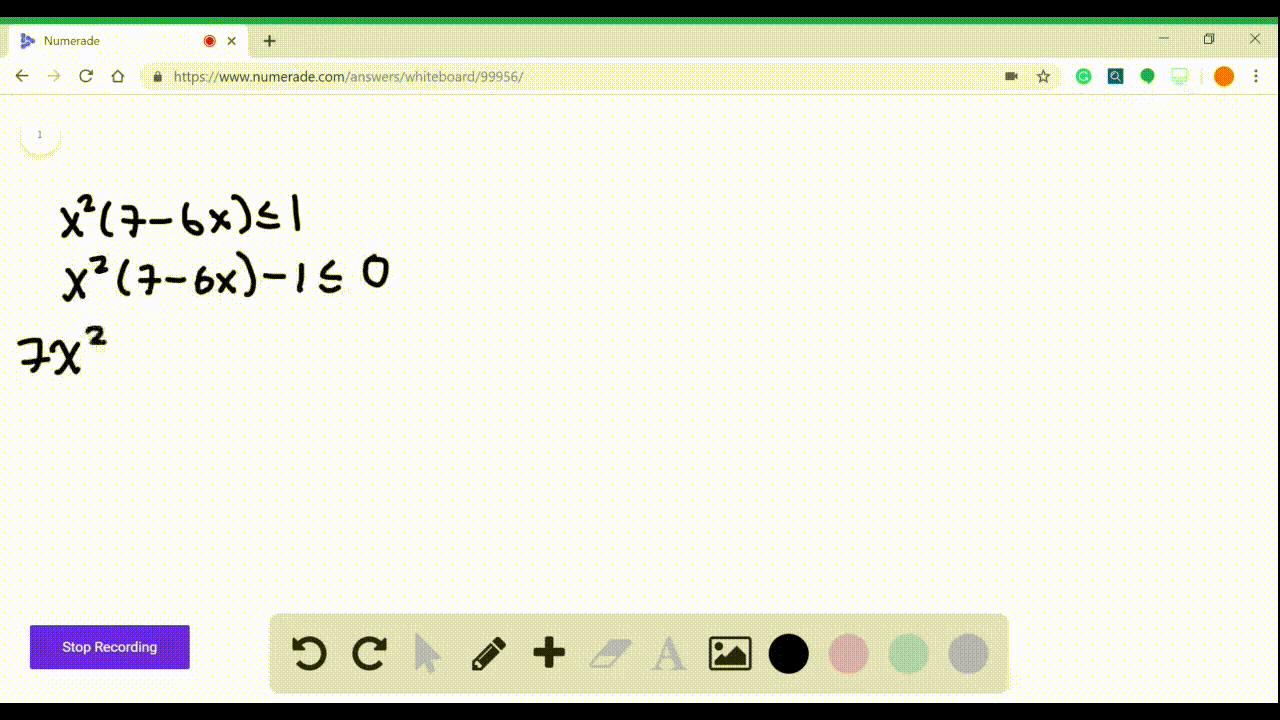Enroll in one of our FREE online STEM summer camps. Space is limited so join now!View Summer Courses### $3-16=$ Polynomial Inequalities Solve the inequal…

08:49Other Schools

Need more help? Fill out this quick form to get professional live tutoring.

Get live tutoring
Problem 15

$3-16=$ Polynomial Inequalities Solve the inequality.
$$x\left(1-x^{2}\right)^{3}>7\left(1-x^{2}\right)^{3}$$

## Discussion

You must be signed in to discuss.

## Video Transcript

we have to solve this inequality ex onto one minus X squared, cubed. It's greater than seven onto one minus X squared, cubed. So what I notice right away is that first of all, I need to move everything on one side. But I also know is that this is exactly the same terms. So I'm gonna bring it over X on the one minus X squared, cubed minus seven under one minus X squared. Cubed is greater than zero and then I'm gonna factor it age. So when there was a right one minus x squared cubed out here and then I'm gonna say what's left. So imagine that I remove this and remove this. I'm left with X minus seven is greater than zero. So that gives me a possible route here of X equals plus or minus one because one minus X squared is equal to zero, which means one equals X squared. So X equals plus or minus one. That's the first option where the 1st 2 options really, and then the other option is acceptable to seven. So my number line that I've produced is going to be have minus one on it one and then seven and then I'm looking at two factors. One minus X squared, cubed and I'm looking at X minus seven. And then I'm looking at the combination of those in Terms of them multiplied together. So I need something less than minus one. So what's a minus to plug in minus two. Here, minus two squared is 41 minus four is negative. Three and negative three cubed is negative. I don't really care what the number is and then minus two minus seven is also negative. So when I combine them, I get a positive. If I plug in zero in here, I get one cube, which is positive, and I get minus seven. Sorry, I don't need the seven part. I just need the negative. So that gives me a negative value in here between minus one and one that if I plug in to, um one minus two squared, cubed is negative again. And that's negative. So it gives me a positive. And then finally, something bigger than seven. That's a eight, gives me a negative and positive, which means it's negative. So when I look over here, I'm looking for where is greater than zero which means I'm looking for the positive places. So I'm looking for here and here. So my solution is going to be from negative infinity up to minus one. That's the first interval and the other interval is the interval from 1 to 7, and that is my answer.